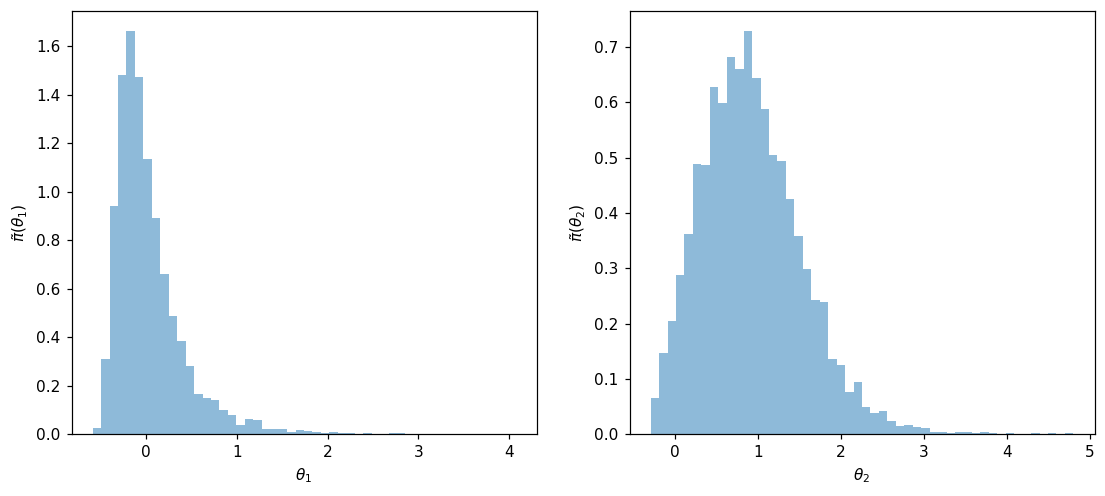Launch this notebook on on mybinder.org:# Characterization of Bayesian posterior density#

The objective of this example is to demonstrate how transport maps can be used to represent posterior distribution of Bayesian inference problems.

## Imports#

First, import MParT and other packages used in this notebook. Note that it is possible to specify the number of threads used by MParT by setting the KOKKOS_NUM_THREADS environment variable before importing MParT.

:

import numpy as np
import scipy
from scipy.optimize import minimize
import matplotlib.pyplot as plt
from scipy.stats import multivariate_normal

import os

import mpart as mt
plt.rcParams['figure.dpi'] = 110

Kokkos is usingKokkos::OpenMP::initialize WARNING: You are likely oversubscribing your CPU cores.

Kokkos::OpenMP::initialize WARNING: You are likely oversubscribing your CPU cores.
Detected: 2 cores per node.
Detected: 1 MPI_ranks per node.


## Problem formulation#

### Bayesian inference#

A way construct a transport map is from an unnormalized density. One situation where we know the probality density function up to a normalization constant is when modeling inverse problems with Bayesian inference.

For an inverse problem, the objective is to characterize the value of some parameters $$\boldsymbol{\theta}$$ of a given system, knowing some the value of some noisy observations $$\mathbf{y}$$.

With Bayesian inference, the characterization of parameters $$\boldsymbol{\theta}$$ is done via a posterior density $$\pi(\boldsymbol{\theta}|\mathbf{y})$$. This density characterizes the distribution of the parameters knowing the value of the observations.

Using Bayes’ rule, the posterior can decomposed into the product of two probability densities:

1. The prior density $$\pi(\boldsymbol{\theta})$$ which is used to enforce any a priori knowledge about the parameters.

2. The likelihood function $$\pi(\mathbf{y}|\boldsymbol{\theta})$$. This quantity can be seen as a function of $$\boldsymbol{\theta}$$ and gives the likelihood that the considered system produced the observation $$\mathbf{y}$$ for a fixed value of $$\boldsymbol{\theta}$$. When the model that describes the system is known in closed form, the likelihood function is also knwon in closed form.

$\pi(\boldsymbol{\theta}|\mathbf{y}) = \frac{1}{c} \pi(\mathbf{y}|\boldsymbol{\theta}) \pi(\boldsymbol{\theta})$

where $$c = \int \pi(\mathbf{y}|\boldsymbol{\theta}) \pi(\boldsymbol{\theta}) d\theta$$ is an normalizing constant that ensures that the product of the two quantities is a proper density. Typically, the integral in this definition cannot be evaluated and $$c$$ is assumed to be unknown.

The objective of this examples is, from the knowledge of $$\pi(\mathbf{y}|\boldsymbol{\theta})\pi(\boldsymbol{\theta})$$ build a transport map that transports samples from the reference $$\eta$$ to samples from posterior $$\pi(\boldsymbol{\theta}|\mathbf{y})$$.

### Application with the Biochemical Oxygen Demand (BOD) model from [Sullivan et al., 2010]#

#### Definition#

To illustrate the process describe above, we consider the BOD inverse problem described in [Marzouk et al., 2016]. The goal is to estimate $$2$$ coefficients in a time-dependent model of oxygen demand, which is used as an indication of biological activity in a water sample.

The time dependent forward model is defined as

$\mathcal{B}(t) = A(1-\exp(-Bt))+\mathcal{E},$

where

\begin{split}\begin{aligned} \mathcal{E} & \sim \mathcal{N}(0,1e-3)\\ A & = \left[0.4 + 0.4\left(1 + \text{erf}\left(\frac{\theta_1}{\sqrt{2}} \right)\right) \right]\\ B & = \left[0.01 + 0.15\left(1 + \text{erf}\left(\frac{\theta_2}{\sqrt{2}} \right)\right) \right] \end{aligned}\end{split}

The objective is to characterize the posterior density of parameters $$\boldsymbol{\theta}=(\theta_1,\theta_2)$$ knowing observation of the system at time $$t=\left\{1,2,3,4,5 \right\}$$ i.e. $$\mathbf{y} = (y_1,y_2,y_3,y_4,y_5) = (\mathcal{B}(1),\mathcal{B}(2),\mathcal{B}(3),\mathcal{B}(4),\mathcal{B}(5))$$.

Definition of the forward model and gradient with respect to $$\mathbf{\theta}$$:

:

def forward_model(p1,p2,t):
A = 0.4+0.4*(1+scipy.special.erf(p1/np.sqrt(2)))
B = 0.01+0.15*(1+scipy.special.erf(p2/np.sqrt(2)))
out = A*(1-np.exp(-B*t))
return out

A = 0.4+0.4*(1+scipy.special.erf(p1/np.sqrt(2)))
B = 0.01+0.15*(1+scipy.special.erf(p2/np.sqrt(2)))
dBdx2 = 0.119683*np.exp(-0.5*p2**2)
dOutdx2 = t*A*dBdx2*np.exp(-t*B)
return np.vstack((dOutdx1,dOutdx2))


One simulation of the forward model:

:

t = np.linspace(0,10, 100)
fig, ax = plt.subplots(1,1)
ax.plot(t, forward_model(1, 1, t));
ax.set_xlabel("t")
ax.set_ylabel("BOD");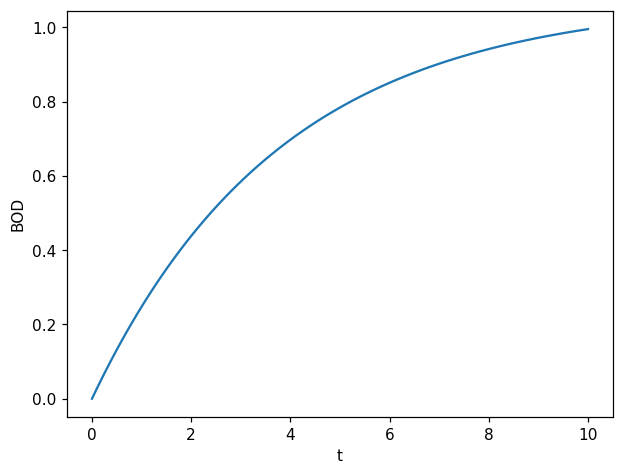For this problem, as noise $$\mathcal{E}$$ is Gaussian and additive, the likelihood function $$\pi(\mathbf{y}|\boldsymbol{\theta})$$, can be decomposed for each time step as:

$\pi(\mathbf{y}|\boldsymbol{\theta}) = \prod_{t=1}^{5} \pi(y_t|\boldsymbol{\theta}),$

where

$\pi(\mathbf{y}_t|\boldsymbol{\theta})=\frac{1}{\sqrt{0.002.\pi}}\exp \left(-\frac{1}{0.002} \left(y_t - \mathcal{B}(t)\right)^2 \right), t \in \{1,...,5\}.$

Likelihood function and its gradient with respect to parameters:

:

def log_likelihood(std_noise,t,yobs,p1,p2):
y = forward_model(p1,p2,t)
log_lkl = -np.log(np.sqrt(2*np.pi)*std_noise)-0.5*((y-yobs)/std_noise)**2
return log_lkl

y = forward_model(p1,p2,t)


We can then define the unnormalized posterior and its gradient with respect to parameters:

:

def log_posterior(std_noise,std_prior1,std_prior2,list_t,list_yobs,p1,p2):
log_prior1 = -np.log(np.sqrt(2*np.pi)*std_prior1)-0.5*(p1/std_prior1)**2
log_prior2 = -np.log(np.sqrt(2*np.pi)*std_prior2)-0.5*(p2/std_prior2)**2
log_posterior = log_prior1+log_prior2
for k,t in enumerate(list_t):
log_posterior += log_likelihood(std_noise,t,list_yobs[k],p1,p2)
return log_posterior

for k,t in enumerate(list_t):


#### Observations#

We consider the following realization of observation $$\mathbf{y}$$:

:

list_t = np.array([1,2,3,4,5])
list_yobs = np.array([0.18,0.32,0.42,0.49,0.54])

std_noise = np.sqrt(1e-3)
std_prior1 = 1
std_prior2 = 1


#### Visualization of the unnormalized posterior density#

:

Ngrid = 100
x = np.linspace(-0.5, 1.25, Ngrid)
y = np.linspace(-0.5, 2.5, Ngrid)
X, Y = np.meshgrid(x, y)

Z = log_posterior(std_noise,std_prior1,std_prior2,list_t,list_yobs,X.flatten(),Y.flatten())
Z = np.exp(Z.reshape(Ngrid,Ngrid))

fig, ax = plt.subplots()
CS = ax.contour(X, Y, Z)
ax.set_xlabel(r'$\theta_1$')
ax.set_ylabel(r'$\theta_2$')
plt.show()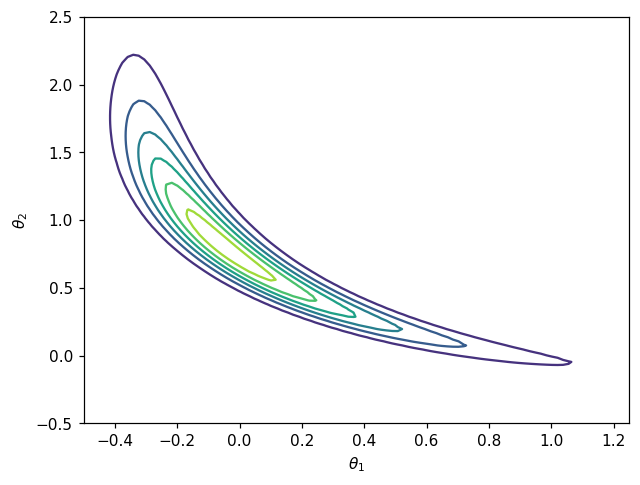Target density for the map from density is non-Gaussian which mean that a non linear map will be required to achieve good approximation.

## Map computation#

After the definition of the log-posterior and gradient, the construction of the desired map $$T$$ to characterize the posterior density result in a “classic” map from unnomarlized computation.

### Definition of the objective function:#

Knowing the closed form of unnormalized posterior $$\bar{\pi}(\boldsymbol{\theta} |\mathbf{y})= \pi(\mathbf{y}|\boldsymbol{\theta})\pi(\boldsymbol{\theta})$$, the objective is to find a map-induced density $$\tilde{\pi}_{\mathbf{w}}(\mathbf{x})$$ that is a good approximation to the posterior $$\pi(\boldsymbol{\theta} |\mathbf{y})$$.

In order to characterize this posterior density, one method is to build a transport map.

For the map from unnormalized density estimation, the objective function on parameter $$\mathbf{w}$$ reads:

$J(\mathbf{w}) = - \frac{1}{N}\sum_{i=1}^N \left( \log\pi\left(T(\mathbf{z}^i;\mathbf{w})\right) + \log \text{det }\nabla_\mathbf{z} T(\mathbf{z}^i;\mathbf{w})\right), \,\,\, \mathbf{z}^i \sim \mathcal{N}(\mathbf{0},\mathbf{I}_d),$

where $$T$$ is the transport map pushing forward the standard normal $$\mathcal{N}(\mathbf{0},\mathbf{I}_d)$$ to the target density $$\pi(\mathbf{x})$$, which will be the the posterior density. The gradient of this objective function reads:

$\nabla_\mathbf{w} J(\mathbf{w}) = - \frac{1}{N}\sum_{i=1}^N \left( \nabla_\mathbf{w} T(\mathbf{z}^i;\mathbf{w}).\nabla_\mathbf{x}\log\pi\left(T(\mathbf{z}^i;\mathbf{w})\right) + \nabla_{\mathbf{w}}\log \text{det }\nabla_\mathbf{z} T(\mathbf{z}^i;\mathbf{w})\right), \,\,\, \mathbf{z}^i \sim \mathcal{N}(\mathbf{0},\mathbf{I}_d).$
:

def grad_x_log_target(x):
return out

def log_target(x):
out = log_posterior(std_noise,std_prior1,std_prior2,list_t,list_yobs,x[0,:],x[1,:])
return out

def obj(coeffs, tri_map,x):
num_points = x.shape
tri_map.SetCoeffs(coeffs)
map_of_x = tri_map.Evaluate(x)
rho_of_map_of_x = log_target(map_of_x)
log_det = tri_map.LogDeterminant(x)
return -np.sum(rho_of_map_of_x + log_det)/num_points

num_points = x.shape
tri_map.SetCoeffs(coeffs)
map_of_x = tri_map.Evaluate(x)

:

#Draw reference samples to define objective
N=10000
z = np.random.randn(2,N)


#### Map parametrization#

We use the MParT function CreateTriangular to directly create a transport map object of dimension with given total order parameterization.

:

# Create transport map
opts = mt.MapOptions()
total_order = 3
tri_map = mt.CreateTriangular(2,2,total_order,opts)


#### Optimization#

:

options={'gtol': 1e-2, 'disp': True}
res = scipy.optimize.minimize(obj, tri_map.CoeffMap(), args=(tri_map, z), jac=grad_obj, method='BFGS', options=options)

Optimization terminated successfully.
Current function value: -6.225731
Iterations: 19
Function evaluations: 23


## Accuracy checks#

### Comparing density contours#

Comparison between contours of the posterior $$\pi(\boldsymbol{\theta}|\mathbf{y})$$ and conoturs of pushforward density $$T_\sharp \eta$$.

:

# Pushforward distribution
def push_forward_pdf(tri_map,ref,x):
xinv = tri_map.Inverse(x,x)
return np.exp(log_pdf)

:

# Reference distribution
ref = multivariate_normal(np.zeros(2),np.eye(2))

xx_eval = np.vstack((X.flatten(),Y.flatten()))
Z2 = push_forward_pdf(tri_map,ref,xx_eval)
Z2 = Z2.reshape(Ngrid,Ngrid)

fig, ax = plt.subplots()
CS1 = ax.contour(X, Y, Z)
CS2 = ax.contour(X, Y, Z2,linestyles='dashed')
ax.set_xlabel(r'$\theta_1$')
ax.set_ylabel(r'$\theta_2$')
h1,_ = CS1.legend_elements()
h2,_ = CS2.legend_elements()
ax.legend([h1, h2], ['Unnormilzed posterior', 'TM approximation'])
plt.show()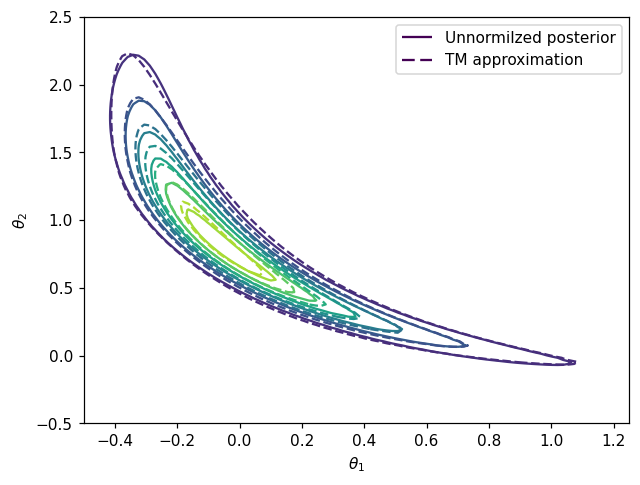### Variance diagnostic#

A commonly used accuracy check when facing computation maps from density is the so-called variance diagnostic defined as:

$\epsilon_\sigma = \frac{1}{2} \mathbb{V}\text{ar}_\rho \left[ \log \frac{\rho}{T^\sharp \bar{\pi}} \right]$

This diagnostic is asymptotically equivalent to the minimized KL divergence $$D_{KL}(\eta || T^\sharp \pi)$$ and should converge to zero when the computed map converge to the theoritical true map.

:

def variance_diagnostic(tri_map,ref,target_logpdf,x):
ref_logpdf = ref.logpdf(x.T)
y = tri_map.Evaluate(x)
pullback_logpdf = target_logpdf(y) + tri_map.LogDeterminant(x)
diff = ref_logpdf - pullback_logpdf
expect = np.mean(diff)
var = 0.5*np.mean((diff-expect)**2)
return var

:

# Reference distribution
ref_distribution = multivariate_normal(np.zeros(2),np.eye(2));

test_z = np.random.randn(2,10000)
# Compute variance diagnostic
var_diag = variance_diagnostic(tri_map,ref,log_target,test_z)

# Print final coeffs and objective
print('==================')
print('Variance diagnostic: {:.2E}'.format(var_diag))
print('==================')

==================
Variance diagnostic: 2.57E-02
==================


### Drawing samples from approximate posterior#

Once the transport map from reference to unnormalized posterior is estimated it can be used to sample from the posterior to characterize the Bayesian inference solution.

:

Znew = np.random.randn(2,5000)
colors = np.arctan2(Znew[1,:],Znew[0,:])

Xpost = tri_map.Evaluate(Znew)

fig,axs = plt.subplots(ncols=2,figsize=(12,5))
axs.scatter(Xpost[0,:],Xpost[1,:], c=colors, alpha=0.2)
axs.set_aspect('equal', 'box')
axs.set_xlabel(r'$\theta_1$')
axs.set_ylabel(r'$\theta_2$')
axs.set_title('Approximate Posterior Samples')

axs.scatter(Znew[0,:],Znew[1,:], c=colors, alpha=0.2)
axs.set_aspect('equal', 'box')
axs.set_xlabel(r'$z_1$')
axs.set_ylabel(r'$z_2$')
axs.set_title('Reference Samples')

plt.show()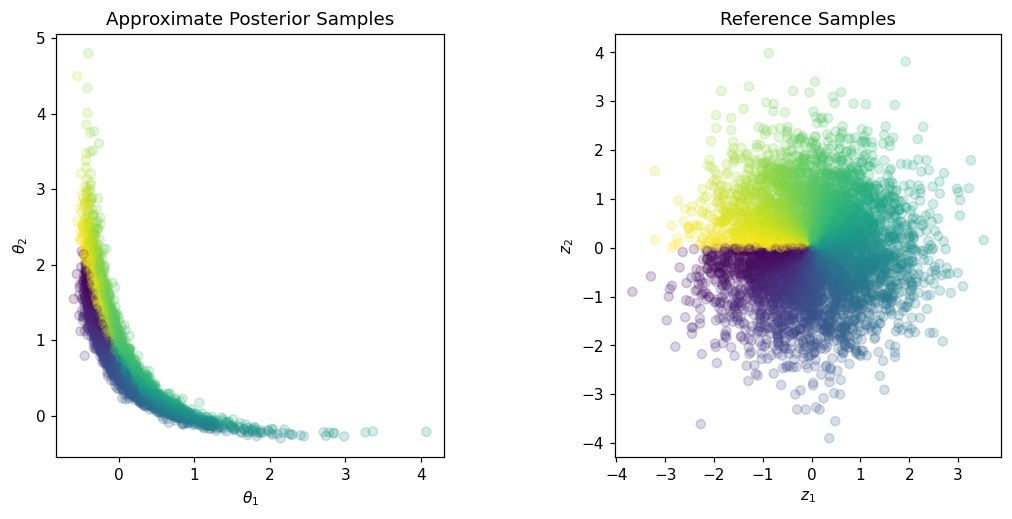Samples can then be used to compute quantity of interest with respect to parameters $$\boldsymbol{\theta}$$. For example the sample mean:

:

X_mean = np.mean(Xpost,1)
print('Mean a posteriori: '+str(X_mean))

Mean a posteriori: [0.01588626 0.95063135]


Samples can also be used to study parameters marginas. Here are the one-dimensional marginals histograms:

:

fig, ax = plt.subplots(1,2,figsize=(12,5))
ax.hist(Xpost[0,:], 50, alpha=0.5, density=True)
ax.set_xlabel(r'$\theta_1$')
ax.set_ylabel(r'$\tilde{\pi}(\theta_1)$')
ax.hist(Xpost[1,:], 50, alpha=0.5, density=True)
ax.set_xlabel(r'$\theta_2$')
ax.set_ylabel(r'$\tilde{\pi}(\theta_2)$')
plt.show()